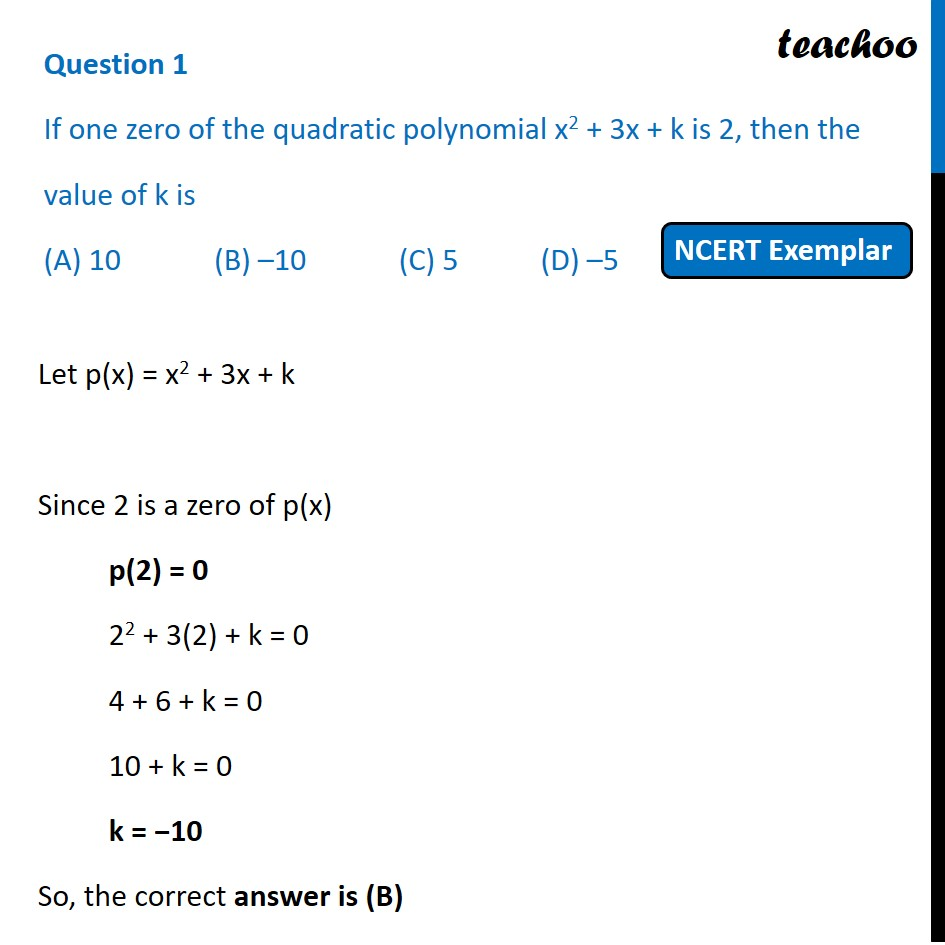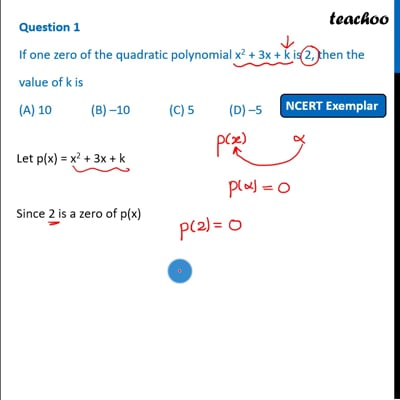MCQs from NCERT Exemplar

Chapter 2 Class 10 Polynomials
Serial order wise

## (A) 10       (B) –10             (C) 5             (D) –5This video is only available for Teachoo black users

Introducing your new favourite teacher - Teachoo Black, at only ₹83 per month

### Transcript

Question 1 If one zero of the quadratic polynomial x2 + 3x + k is 2, then the value of k is (A) 10 (B) –10 (C) 5 (D) –5 Let p(x) = x2 + 3x + k Since 2 is a zero of p(x) p(2) = 0 22 + 3(2) + k = 0 4 + 6 + k = 0 10 + k = 0 k = −10 So, the correct answer is (B)